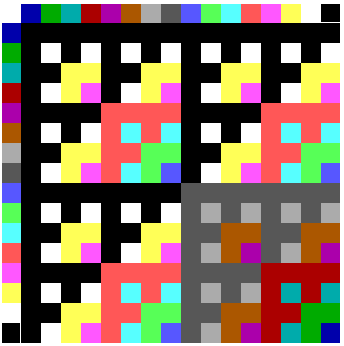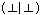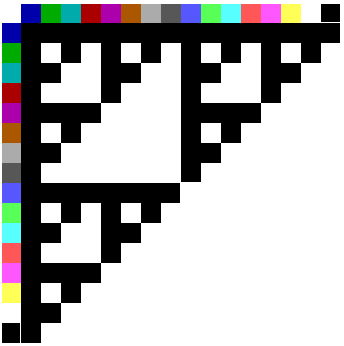5. The Sierpinski Tautology Map

In this section we outline another way of visualizing simple formal systems, which to our surprise turned out to offer intriguing links to classic patterns in fractal geometry.

In the rug patterns above we graphed an enumeration of formulae for simple forms of propositional calculi, coloring the grid locations of formulae in terms of their values. For forms of propositional calculus with n sentence letters, we noted, there will be 2 raised to 2n such colors or values--essentially, a color for each possible truth table of length 2n. Here we consider a different type of display for such systems, constructed using those values themselves on the axes rather than enumerated wffs. This frees us from particular enumerations of formulae because it frees us from the formulae themselves; the value space is constructed not in terms of particular formulae but in terms of the values of equivalence classes of formulae.

Consider two sentence letters p and q in standard truth-table form:

 p q p q T T F F T F T F 1 1 0 0 1 0 1 0

For the four-line truth-tables appropriate to two sentence letters there are sixteen possible combinations of T and F, or 1 and 0. These include solid 0's, corresponding to a contradiction or necessary falsehood; solid 1's, corresponding to a tautology; the pattern 1100, corresponding to the value of p; and the pattern 1010, corresponding to q. The sixteen values for two sentence letters can be thought of simply as all four-digit binaries.Fig. 19

These can be arranged in ascending order along the two axes of a two-dimensional display. Following the approach above we can think of these values as distinguished by color as well (Figure 19).Fig. 20

Combinatorial values for any chosen binary connective can now be mapped in the interior value space. If our value map is that of the Sheffer stroke, for example, the value ofwill appear at the intersection of 0000 and 0000; the value ofat the intersection of 1111 and 1100, etc. In terms of the colors on our axes the complete graph for the Sheffer stroke appears in Figure 20.Fig. 21

It is clear that a Sheffer stroke between and 0000 or any other value amounts to a tautology. In Figure 21, 0000 is represented using the darkest grey, tautologies are shown in black, and the fact just noted is represented by black values representing tautology in all cases along the left column and along the top row--all cases in which a value of 0000 appears on either side of the stroke. A Sheffer stroke between two tautologies, on the other hand, amounts to a contradiction, indicated by the dark grey square at the intersection of two black axis values in the lower right corner. As a whole the graph represents the value space for all Sheffer stroke combinations of our sixteen values.Fig. 22

A particularly intriguing feature of the value space appears more dramatically if we emphasize tautologies in particular by whiting out all other values (Figure 21). The fractal pattern formed here in black is that of the Sierpinski gasket, which has long been a primary exhibit in fractal geometry.3Fig. 23

If we expand our value space to that of three variables, with 256 values corresponding to all eight-digit binaries, an even finer representation of the Sierpinski gasket appears (Figure 22).

At any number of variables, given a standard listing of binaries corresponding to truth-table values, the tautologous Sheffer combinations will form a Sierpinski gasket. As indicated below, we can in fact think of diagrams with increasing numbers of sentence letters as increasingly finer approximations to a full system, with infinitely many sentence letters and infinitely many values. For that diagram, the tautologies of the system would form an infinitely fine Sierpinski dust.

The main connective of Figures 20 through 22 is NAND or the Sheffer stroke. A similar display for NOR, or the dagger, appears in Figure 23. Here there is only one tautology, at the intersection of 0000 and 0000. The Sierpinski gasket does show up again, however, as a graph of contradictions in the lower right-hand corner.

Other connectives generate other patterns in value space. A standard 'and' and 'or', for example, are shown in Figure 24. In the case of 'and' the persistent image of the Sierpinski gasket appears in the upper left as a value pattern for contradiction; in the case of 'or' it appears in the lower right as a value pattern for tautology. In material implication the Sierpinski triangle shifts to the lower left as a value pattern for tautology.Fig. 24

In the course of our research the appearance of the Sierpinski gasket within the value space of propositional logic came as a surprise. But its appearance can easily be understood after the fact.

As indicated above, we can think of value space displays for forms of propositional calculus with increasing numbers of sentence letters as approximations to a fuller system. As long as we have some finite number of sentence letters n we will have finitely many value spaces, corresponding to all possible truth tables of length 2n. But the full propositional calculus has a countably infinite number of sentence letters. Because standard propositional calculus is limited to wffs of finite length, it turns out, we can construct a value space for it using something like truth-tables of infinite length.

This is less difficult than it may at first appear. In constructing finite truth-tables for n variables the standard procedure is to start with a sentence letter represented as 0101... to length 2n , to represent the next sentence letter with 00110011... to that length, the third with 00001111..., and so forth. For infinite truth-tables adequate to finite complexes of countably many sentence letters our first sentence letter p can be thought of as having an infinite truth-table that starts 01010101... . Our second sentence letter q can be thought of as having the infinite truth-table that starts 00110011... , our third sentence letter r as having the infinite truth-table 000011111, and so forth. Each of our sentence letters, in other words, can be thought of as having infinitely periodic truth-tables which otherwise follow the standard scheme used for constructing truth-tables of finite length. There will always be room for 'one more' sentence letter because it will always be possible to introduce a larger period of 0's and 1's for the next sentence- letter needed. Sentence-letters of a full form of propositional calculus can thus be thought of as corresponding to a subset of the periodic binary decimals: those which alternate series of 0's and 1's of length 2n for some n.

Any set of values for any finite set of sentence letters will have an appropriate line in this infinite extension of truth-tables, and in fact will have a line that will itself reappear an infinite number of times. Because the infinite truth-tables for our sentence letters are periodic in this way, complex sentences formed of finitely many connectives between finitely many sentence letters will be periodic as well. The largest period possible for a complex sentence of this sort will in fact be the longest period of its sentence-letter components. All values for the full propositional calculus will thus be represented by periodic binary decimals. It is important to note, however, that not all periodic binary decimals will have a corresponding formula; those periodic in multiples of 3, for example, will not be producible by finite combination from sentence-letters periodic in powers of 2.4

The important point here is simply that any value space for finitely many sentence letters can be thought of as an approximation to this richer system, adequate to propositional calculus as a whole. In the richer system, of course, the squares of the value spaces illustrated above shrink to mere points in value space, just as values on the axes shrink to mere points on the continuum. Although these points do not by any means exhaust the full [0,1] interval--they constitute merely a subset of the periodic decimals--they can be envisaged as embedded within it. The argument below regarding the appearance of the Sierpinski triangle applies to a full continuum as well as the envisaged subsets, and will be valid both for the full propositional calculus and for the envisaged approximations to it.

In terms of NAND or the Sheffer stroke the appearance of the Sierpinski triangle can be outlined as follows. Similar explanations will apply for the other connectives.

Let us emphasize that the binary representations of values on each axis of our value spaces, whether finite or infinite, correspond to columns of a truth-table. The value assigned to any value space or point v is a function of the truth-table values from which it is perpendicular on each axis. In asking whether a point in the value space represents a tautology in a graph for NAND, for example, what we're really asking is whether the truth-tables of these two axis values share any line in which both show a '1'. If there is such a line, their combination by way of NAND is not a tautology. The value point v will have the value of a tautology if and only if its axis values at no point show a '1' on the same line.Fig. 25

Consider now one standard route to the Sierpinski gasket, which generates the gasket from a triangle in terms of a rule for doubling distance from the nearest vertex (Mentioned in Manfred Schroeder's book "Fractals, Chaos, Power Laws"). For any given triangle, there is a set of points which, when distance is doubled from the nearest vertex, will be 'thrown' outside of the triangle itself--more precisely, which will map under doubling from the nearest vertex to points outside the triangle itself. These points in fact form an inverted triangle in the center (Figure 25). There are a further set of points which, when distance is doubled from the nearest vertex, will be thrown into this central region--and thus which will be thrown out of the triangle upon two iterations of the 'doubling from nearest vertex' procedure. The Sierpinski gasket is composed of all those points which will remain within the triangle despite unlimited iteration of such a procedure

. It turns out that this route to the Sierpinski gasket corresponds quite neatly to its appearance as a map of theorems in the value space for NAND.Fig. 26

Consider the diagram of a unit square in Figure 26, and the upper triangle marked between A (0,1), B (0,0), and C (1,0). This 'inverted' form of the unit square corresponds to our axes for value spaces above. Were we to characterize the rule of doubling the distance from the closest vertex in terms of x and y values for particular points within this triangle, our rules could be rendered as follows:

If B is closest, (xn, yn) = (2x, 2y)
If A is closest, (xn, yn) = (2x, 1-2(1-y))
If C is closest, (xn, yn) = (1-2(1-x), 2y)

These will give us the Sierpinski gasket by the standard route of doubling the distance from the nearest vertex.

Here it's clear that 'doubling the distance' is in all cases a matter of either multiplying an axis value by 2 or substracting 1 from a multiplication by 2. But now let us envisage the axes of our unit square as marked in terms of binary decimals. For binary decimals multiplication by 2 simply involves moving a decimal point one place to the right. 1-2(1-x) equals 2x-1, which moves the decimal point one place to the right and 'lops off' any ones that thereby migrate to the left of the decimal point. The crucial point is that for binary decimal expression of axis values, both forms of transform preserve the order of digits which remain beyond the decimal point. Iterated application of such transforms to pairs of values (x,y) thus effectively moves down each series of binary digits one at a time, checking for whether a 1 occurs in both places. If it does, our iterated transforms have resulted in two values both of which are greater than 1/2 as expressed in binary, and the point will therefoer have migrated under iteration outside the region of the dark triangle.

The points of the triangle ABC which will not migrate out under an iterated procedure of doubling the distance from the nearest vertex--the points of a Sierpinski triangle in that upper region--are therefore those points (x,y) such that the binary representation of x and y do not both have a 1 in the same decimal place. Given our representation of values in terms of binary decimals, those points which generate tautologies under NAND will be precisely those same points: points with axis values with no 1's in corresponding truth-table lines, or equivalently with no 1's in corresponding decimal places of their binary representation. The initially startling appearance of the Sierpinski gasket as a map of tautologies under the Sheffer stroke can thus be understood in terms of (i) what a binary representation of values means and (ii) a corresponding rendering of the familiar 'doubling the distance from the nearest vertex' route to the Sierpinski in terms of binaries. An outline for the appearance of the Sierpinski in the value space of other connectives can be drawn along the same lines.

The standard 'distance doubling' route to the Sierpinski does involve a full-continuum unit square. As indicated above, even the full propositonal calculus has a value space short of that full continuum; although each sentence letter and each connective corresponds to an infinite decimal, these form a subset of even the merely periodic decimals. None of that, however, affects the basic mechanism of the argument above, which turns merely on the question of whether two decimals, finite or infinite, share a particular value at any place. Multiplication by 2 from the closest vertex simply 'checks' them place by place. Thus the fact that our value space for the forms of logic at issue constitute mere subsets of the full unit square gives us simply the result that tautologies in the case of NAND, for example, will constitute an infinitely fine Sierpinski dust within that grainy unit square.

One of the promises of a graphic approach to formal systems of this sort is that there may be results of fractal geometry that can be read off as facts about the logical systems at issue. Here the appearance of the Sierpinski gasket as a map of theorems in value space offers a few minor but tantalizing examples.

It is well known that the points constitutive of the Sierpinski gasket within a continuous unit square are infinitely many, but nonetheless 'very few' in the sense that a random selection of points has a probability approaching zero of hitting such a point. The same will be true within the full propositional calculus and its infinitary extension; there will be infinitely many complexes with the value of tautology in such value space, but the probability will approach zero of hitting a tautology in terms of a Sheffer combination of random axis values.

A similar point can be expressed in terms of area. Within any finite approximation to an infinitely fine-grained unit square, the Sierpinski gasket will retain a definite area. Within any value space limited to n sentence-letters, tautologies will retain a similar area of value space. In the case of an infinitely-grained unit square, on the other hand--whether fully continuous or not-- the Sierpinski gasket has an area approaching 0. Within the full propositional calculus the relative area of tautologies will similarly amount to zero. In terms of the Sheffer stroke, tautologies end up distributed as unconnected points within value space on the model of three-dimensional Cantor dust.

For smooth curves, an approxmiate length L(r) can be given as the product of the number N of straight-line segments of length r required to 'cover' the curve from one end to the other. As r goes to zero, L(r) approaches the length of the curve as a finite limit. For fractal curves, on the other hand, it is standard for L(r) to go to infinity as r goes to zero, since what are being 'covered' are increasingly fine parts of the curve. A standard measure d of the intricacy of fractal curves, known as the Hausdorff dimension, is that exponent d such that the product N * rd stays finite. The Hausdorff dimension of the Sierpinski gasket is known to be log3/log21.58. The work above suggests a related value for tautologies within the value space of the Sheffer stroke.